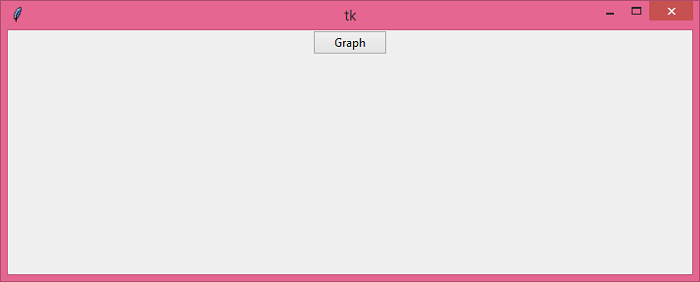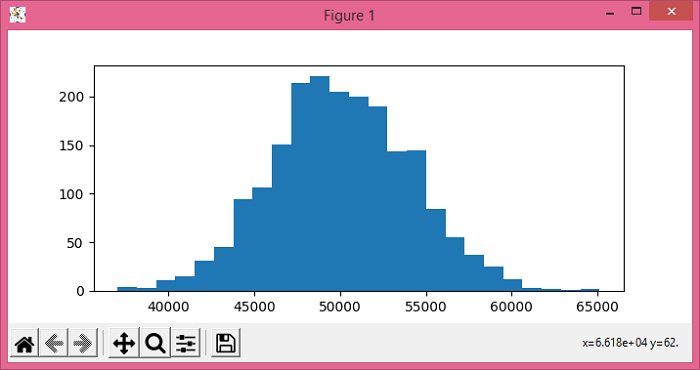# How to run matplotlib in Tkinter?

One of the well-known use-cases of Python is in Machine Learning and Data Science. In order to visualize and plot a dataset, we use the Matplotlib library. To plot a matplotlib graph in a Tkinter application, we have to import the library by initializing "from matplotlib.pyplot as plt". The plot can be drawn either by defining a range value or importing the dataset in the notebook.

## Example

#Import the required Libraries
from tkinter import *
from tkinter import ttk
import numpy as np
import matplotlib.pyplot as plt

#Create an instance of Tkinter frame
win= Tk()

#Set the geometry of the window
win.geometry("700x250")

def graph():
car_prices= np.random.normal(50000,4000,2000)
plt.figure(figsize=(7,3))
plt.hist(car_prices, 25)
plt.show()

#Create a Button to plot the graph
button= ttk.Button(win, text= "Graph", command= graph)
button.pack()

win.mainloop()

## Output

Running the above code will display a window that contains a button.When we click the "Graph" button, it will display a graph on the main window.Updated on: 25-May-2021

480 Views